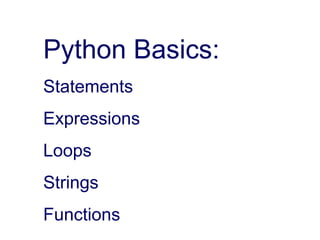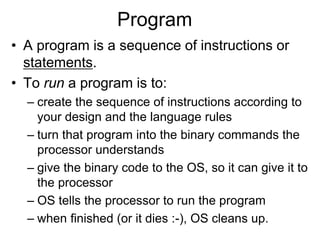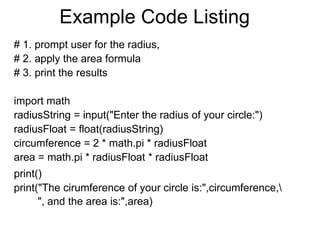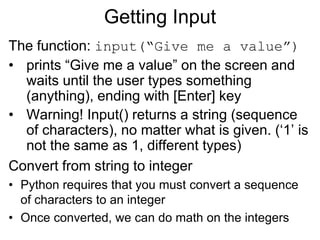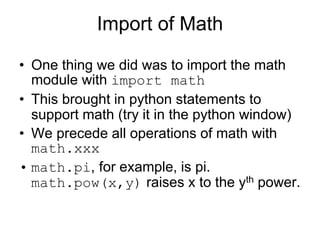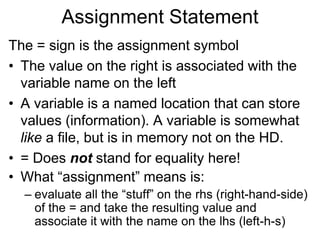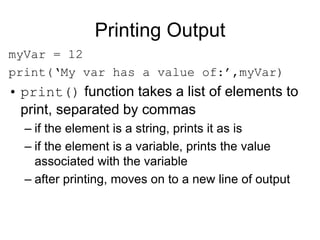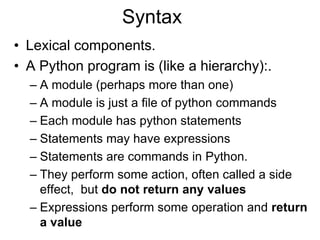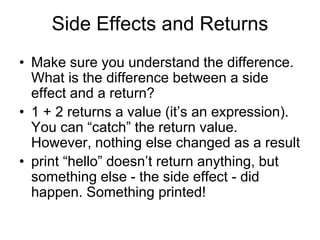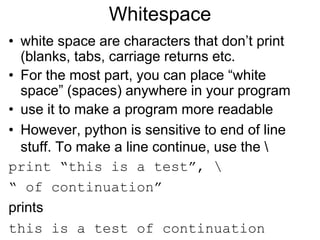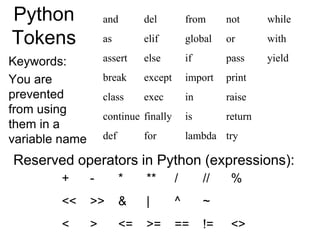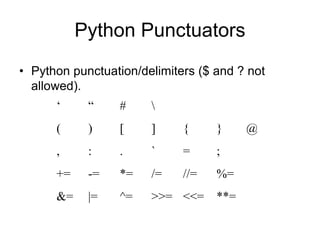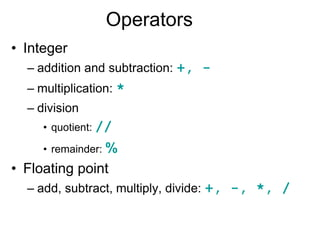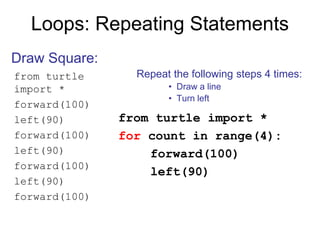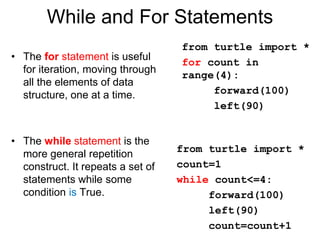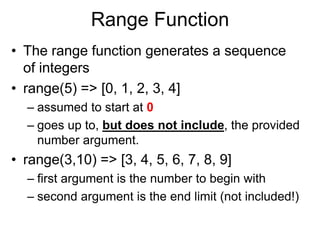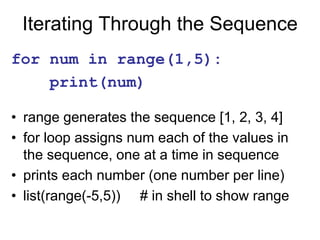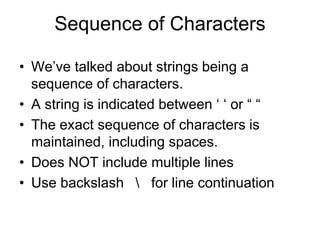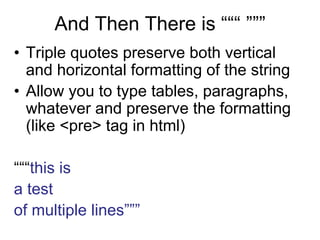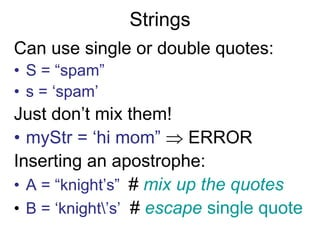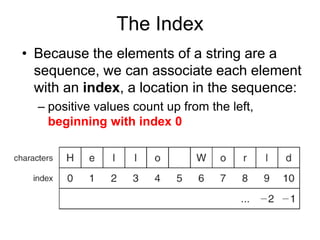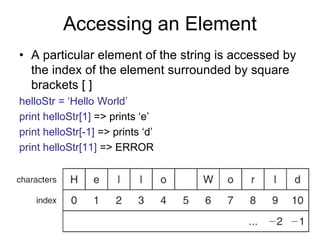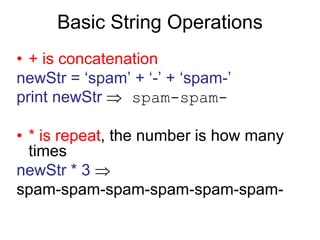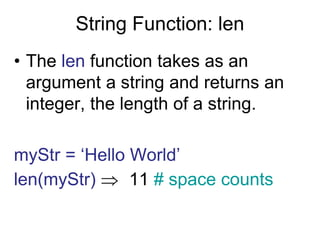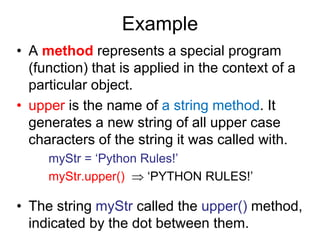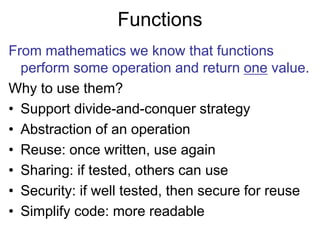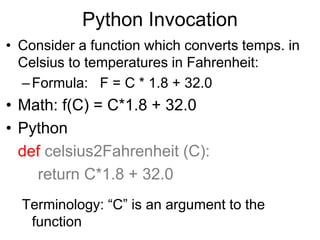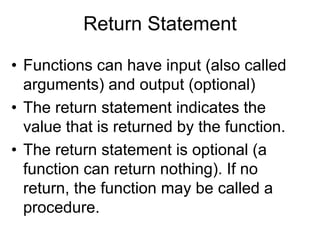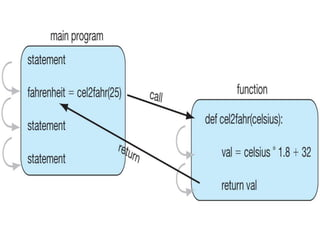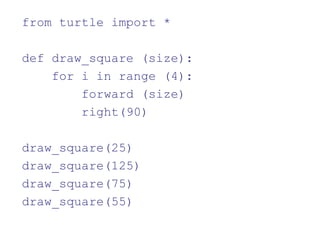1 de 30

### Python basics

1. Python Basics: Statements Expressions Loops Strings Functions
2. Program • A program is a sequence of instructions or statements. • To run a program is to: – create the sequence of instructions according to your design and the language rules – turn that program into the binary commands the processor understands – give the binary code to the OS, so it can give it to the processor – OS tells the processor to run the program – when finished (or it dies :-), OS cleans up.
3. Example Code Listing # 1. prompt user for the radius, # 2. apply the area formula # 3. print the results import math radiusString = input("Enter the radius of your circle:") radiusFloat = float(radiusString) circumference = 2 * math.pi * radiusFloat area = math.pi * radiusFloat * radiusFloat print() print("The cirumference of your circle is:",circumference, ", and the area is:",area)
4. Getting Input The function: input(“Give me a value”) • prints “Give me a value” on the screen and waits until the user types something (anything), ending with [Enter] key • Warning! Input() returns a string (sequence of characters), no matter what is given. (‘1’ is not the same as 1, different types) Convert from string to integer • Python requires that you must convert a sequence of characters to an integer • Once converted, we can do math on the integers
5. Import of Math • One thing we did was to import the math module with import math • This brought in python statements to support math (try it in the python window) • We precede all operations of math with math.xxx • math.pi, for example, is pi. math.pow(x,y) raises x to the yth power.
6. Assignment Statement The = sign is the assignment symbol • The value on the right is associated with the variable name on the left • A variable is a named location that can store values (information). A variable is somewhat like a file, but is in memory not on the HD. • = Does not stand for equality here! • What “assignment” means is: – evaluate all the “stuff” on the rhs (right-hand-side) of the = and take the resulting value and associate it with the name on the lhs (left-h-s)
7. Printing Output myVar = 12 print(‘My var has a value of:’,myVar) • print() function takes a list of elements to print, separated by commas – if the element is a string, prints it as is – if the element is a variable, prints the value associated with the variable – after printing, moves on to a new line of output
8. Syntax • Lexical components. • A Python program is (like a hierarchy):. – A module (perhaps more than one) – A module is just a file of python commands – Each module has python statements – Statements may have expressions – Statements are commands in Python. – They perform some action, often called a side effect, but do not return any values – Expressions perform some operation and return a value
9. Side Effects and Returns • Make sure you understand the difference. What is the difference between a side effect and a return? • 1 + 2 returns a value (it’s an expression). You can “catch” the return value. However, nothing else changed as a result • print “hello” doesn’t return anything, but something else - the side effect - did happen. Something printed!
10. Whitespace • white space are characters that don’t print (blanks, tabs, carriage returns etc. • For the most part, you can place “white space” (spaces) anywhere in your program • use it to make a program more readable • However, python is sensitive to end of line stuff. To make a line continue, use the print “this is a test”, “ of continuation” prints this is a test of continuation
11. Python Tokens Keywords: You are prevented from using them in a variable name and del from not while as elif global or with assert else if pass yield break except import print class exec in raise continue finally is return def for lambda try Reserved operators in Python (expressions): + - * ** / // % << >> & | ^ ~ < > <= >= == != <>
12. Python Punctuators • Python punctuation/delimiters (\$ and ? not allowed). ‘ “ # ( ) [ ] { } @ , : . ` = ; += -= *= /= //= %= &= |= ^= >>= <<= **=
13. Operators • Integer – addition and subtraction: +, - – multiplication: * – division • quotient: // • remainder: % • Floating point – add, subtract, multiply, divide: +, -, *, /
14. Loops: Repeating Statements from turtle import * forward(100) left(90) forward(100) left(90) forward(100) left(90) forward(100) Draw Square: Repeat the following steps 4 times: • Draw a line • Turn left from turtle import * for count in range(4): forward(100) left(90)
15. While and For Statements • The for statement is useful for iteration, moving through all the elements of data structure, one at a time. • The while statement is the more general repetition construct. It repeats a set of statements while some condition is True. from turtle import * for count in range(4): forward(100) left(90) from turtle import * count=1 while count<=4: forward(100) left(90) count=count+1
16. Range Function • The range function generates a sequence of integers • range(5) => [0, 1, 2, 3, 4] – assumed to start at 0 – goes up to, but does not include, the provided number argument. • range(3,10) => [3, 4, 5, 6, 7, 8, 9] – first argument is the number to begin with – second argument is the end limit (not included!)
17. Iterating Through the Sequence for num in range(1,5): print(num) • range generates the sequence [1, 2, 3, 4] • for loop assigns num each of the values in the sequence, one at a time in sequence • prints each number (one number per line) • list(range(-5,5)) # in shell to show range
18. Sequence of Characters • We’ve talked about strings being a sequence of characters. • A string is indicated between ‘ ‘ or “ “ • The exact sequence of characters is maintained, including spaces. • Does NOT include multiple lines • Use backslash for line continuation
19. And Then There is “““ ””” • Triple quotes preserve both vertical and horizontal formatting of the string • Allow you to type tables, paragraphs, whatever and preserve the formatting (like <pre> tag in html) “““this is a test of multiple lines”””
20. Strings Can use single or double quotes: • S = “spam” • s = ‘spam’ Just don’t mix them! • myStr = ‘hi mom”  ERROR Inserting an apostrophe: • A = “knight’s” # mix up the quotes • B = ‘knight’s’ # escape single quote
21. The Index • Because the elements of a string are a sequence, we can associate each element with an index, a location in the sequence: – positive values count up from the left, beginning with index 0
22. Accessing an Element • A particular element of the string is accessed by the index of the element surrounded by square brackets [ ] helloStr = ‘Hello World’ print helloStr => prints ‘e’ print helloStr[-1] => prints ‘d’ print helloStr => ERROR
23. Basic String Operations • + is concatenation newStr = ‘spam’ + ‘-’ + ‘spam-’ print newStr  spam-spam- • * is repeat, the number is how many times newStr * 3  spam-spam-spam-spam-spam-spam-
24. String Function: len • The len function takes as an argument a string and returns an integer, the length of a string. myStr = ‘Hello World’ len(myStr)  11 # space counts
25. Example • A method represents a special program (function) that is applied in the context of a particular object. • upper is the name of a string method. It generates a new string of all upper case characters of the string it was called with. myStr = ‘Python Rules!’ myStr.upper()  ‘PYTHON RULES!’ • The string myStr called the upper() method, indicated by the dot between them.
26. Functions From mathematics we know that functions perform some operation and return one value. Why to use them? • Support divide-and-conquer strategy • Abstraction of an operation • Reuse: once written, use again • Sharing: if tested, others can use • Security: if well tested, then secure for reuse • Simplify code: more readable
27. Python Invocation • Consider a function which converts temps. in Celsius to temperatures in Fahrenheit: –Formula: F = C * 1.8 + 32.0 • Math: f(C) = C*1.8 + 32.0 • Python def celsius2Fahrenheit (C): return C*1.8 + 32.0 Terminology: “C” is an argument to the function
28. Return Statement • Functions can have input (also called arguments) and output (optional) • The return statement indicates the value that is returned by the function. • The return statement is optional (a function can return nothing). If no return, the function may be called a procedure.
29. from turtle import * def draw_square (size): for i in range (4): forward (size) right(90) draw_square(25) draw_square(125) draw_square(75) draw_square(55)